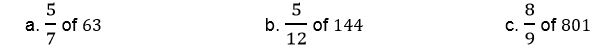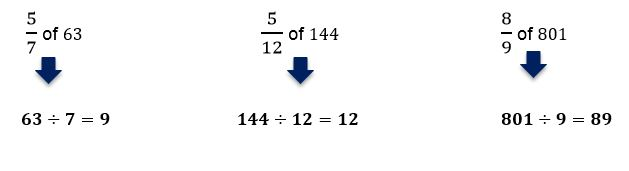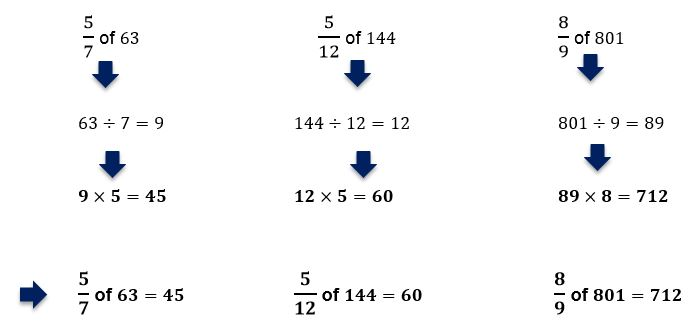# How to work out fractions of amounts

Want to find out how to work out fractions of amounts? We've provided a step-by-step guide of how to work out fractions of amounts to teach you everything you need to know.## Step 1: Divide by the fraction’s denominator

Given the fraction and the amount, divide the amount by the fraction’s denominator. So, if we want to determine 3/5 of 45, we divide 45 by 5.

## Step 2: Multiply the quotient by the numerator

Multiply the quotient by the numerator of the given fraction. This means that 3/5 of 45 is equal to 27.

## Examples of how to work out fractions of amounts

Q1) Work out the following fractions of amounts.To find the fractions of amounts, we first divide the amounts by their corresponding fractions’ denominators.Then multiply the quotient with the fractions’ numerators. Hence, we have the following values below.Q2) Nathaniel received \$210 from his aunt for his birthday. He plans to spend 11/14 of it on new video games. How much is he planning to spend on games?

To find 11/14 of \$210, we first divide the total amount by the denominator. 210÷14=15

Multiply the quotient by 11. Hence, total amount would be

15×11=165

This means that Nathaniel plans to spend \$165 on video games.

Q3) Danny plans to invest 2/15 of his salary every month. If he earns \$5460 every month, how much does he spend monthly?

To determine the amount that he plans to invest monthly, divide the amount of the salary by the fraction’s denominator.

5460÷15=364

Multiply 364 by the given numerator of the fraction.

364×2=728

Danny plans to invest \$728 every month.

#### Join today

The fastest way to practice

Unlock our complete testing platform and improve faster that ever.